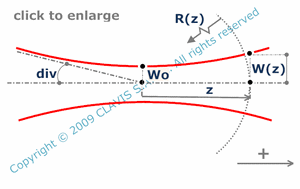and orlaser
gaussian or embedded gaussian beam

# parameters of a gaussian beam ( free )

Caution
Fill in all input cells.
Choose all parameters unit.
Except z and R(z) which are algebric, parameters are strictly positive.
Positive direction is according the horizontal arrow in the bottom right of the scheme.

InputValueunit
wo
z
lam
OutputValueUnit
zoto be calculated
w(z)to be calculated
divto be calculated
R(z)to be calculatedwo : waist radius z : distance from the waist lam : wavelength w(z) : beam radius at a distance z from the waist div : half divergence of the beam R(z) : wavefront radius at a distance z from the waist

Note
This calculation page can not detect all unrealistic inputs.